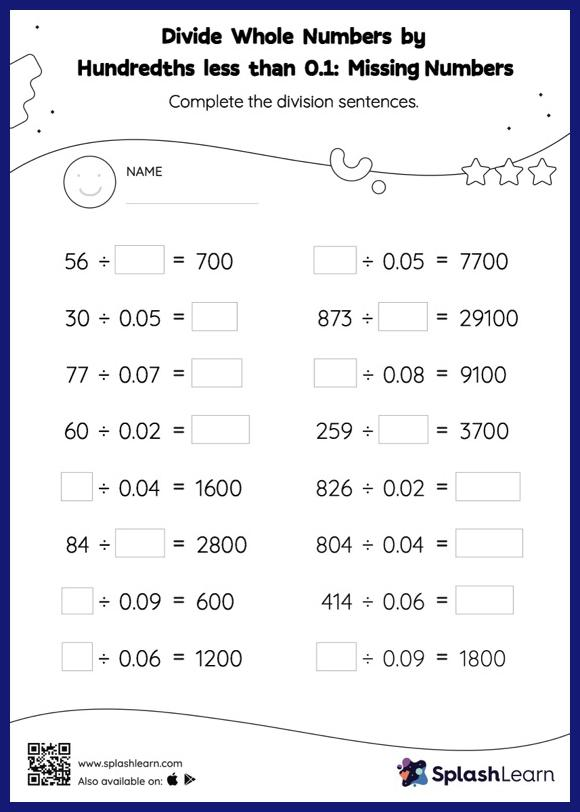# Divide Whole Numbers by Hundredths less than 0.1 without Remainder: Missing Numbers Worksheet

Home > Divide Whole Numbers by Hundredths less than 0.1 without Remainder: Missing NumbersStudents divide a whole number by a decimal without taking the decimal point into account, and then, depending on the number of decimal digits in the divisor, they move each digit of the quotient to the left. They apply this concept in finding the missing number in divide whole numbers by hundredths less than 0.1 Without remainder worksheet. This worksheet is about practicing with the horizontal format in which numbers are written side by side. To develop flexibility with numbers and operations, students need to have enough practice in this format and not just rely on the vertical/column method.